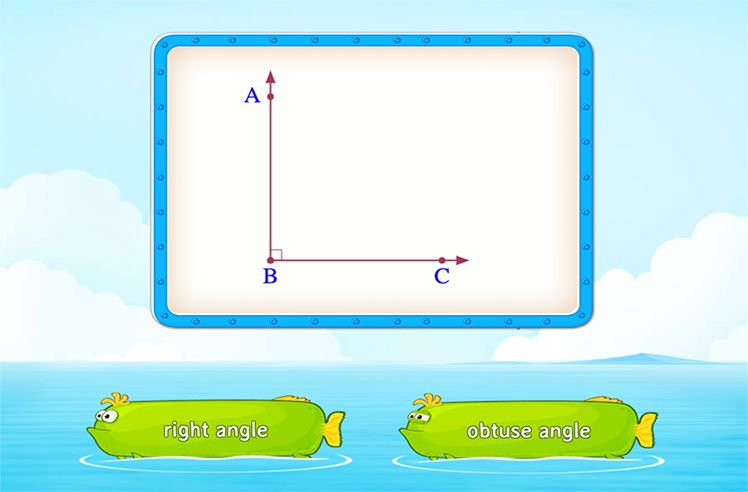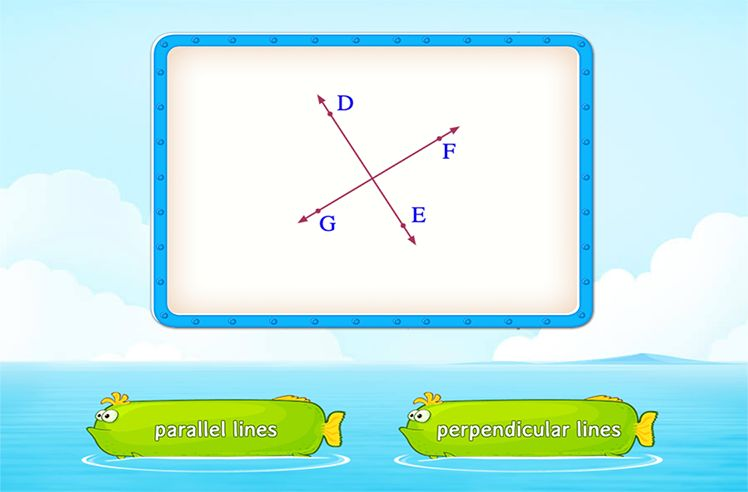# Horizontal Line – Definition with Examples

» Horizontal Line – Definition with Examples

## What is a Horizontal Line?

Imagine you have a paper, and someone asks you to draw a line. What all possibilities do you think will cross your mind? Well; there are a few ways you could do this.

If you were bored; you would most probably just end up drawing a random motion like this; called a skew line.

And if you wanted to think like a mathematician or an artist; you would imagine a tree or something standing and draw a line top to bottom like this; called a vertical line.

Or you could think of a car or something lying down and sketch your line from left to right like this; called a horizontal line.

A horizontal line is a sleeping line. It is a straight line that goes from left to right or right to left. The word horizontal is derived from the word “horizon” which is the line where the earth and sky appear to be meeting far far away.

Horizontal Lines around Us

We see horizontal lines all around us in shapes, objects, figures, and drawings.

Here are some examples of horizontal lines.

Horizontal lines in objects:

## Horizontal Lines in Math:

Horizontal lines are the building blocks of so many 2D and 3D shapes in elementary geometry. Squares, Triangles, Rectangles, Cubes, Trapezoids, Prism are just some basic examples of shapes where we can observe horizontal lines.

Horizontal lines in Coordinate Plane

In coordinate geometry, horizontal lines are defined as lines parallel to the x-axis. If two points on a line have the same y-coordinate points then the line is said to be horizontal.

In the given image AB is a horizontal line parallel to the x-axis and if you observe carefully the y-coordinate of both points A and B are the same. Another interesting this to note about the horizontal lines is that they are always perpendicular to the vertical lines.

Drawing horizontal line

Using a Ruler:

To draw a horizontal line using a ruler, keep the ruler in sleeping position, parallel to the edge of the page and draw the line from left to right or right to left.

To draw a horizontal line on a coordinate plane

• Plot any point on the coordinate plane, for example, (1, 2).
• Identify the y-coordinate of the point marked. Here, it is 2.
• Plot another point on the coordinate plane such that its y-coordinate is the same as the previous point plotted. For example, (–5, 2).
• Join the two points plotted using a ruler to get a horizontal line.

## Uses of Horizontal Lines

Horizontal lines are used in symmetry and photography.

A horizontal line of symmetry runs across the image from left to right (or vice versa) and divides the image into identical halves.

Cameras are designed to take photographs in landscape (horizontal) or portrait (vertical) mode. In horizontal mode, photographs are wider than they are tall. In vertical mode, photographs are taller than they are wide. Our eyes see the surrounding horizontally giving a wider view instead of taller.

Horizontal Line and Vertical Line

## Solved Examples

Example 1: Which line segments represent horizontal lines?

Solution:

Lines BC and AD are horizontal lines.

Example 2: Identify the lines indicated by the arrows in each of the following shapes.

Solution:

1. Horizontal line
2. Vertical line
3. Horizontal line
4. Vertical line

Example 3: Identify the horizontal lines in the given shape.

Solution:

AB, EF, and CD are the horizontal lines in the given shapes.

Example 4: Name five English alphabets that have horizontal lines.

Solution:

E, F, H, L, T

## Practice Problems

A horizontal line is a straight line that is parallel to the x-axis and where all the points on the line have the same y-coordinate.

Yes, all horizontal lines are parallel to each other. Horizontal lines when extended also never intersect with one another.

Horizontal lines are lines that run from left to right and are parallel to the x-axis. Vertical lines are lines that run up and down and are parallel to the y-axis.Shapes

Play NowAngles

Play NowParallel and Perpendicular Lines

Play Now